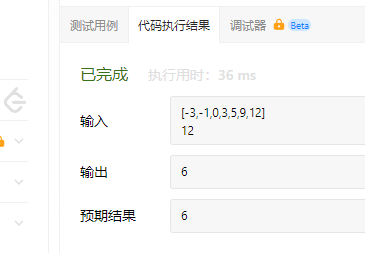# 【每日一题20220726】二分查找‍ 给定一个 n 个元素有序的（升序）整型数组 nums 和一个目标值 target ，写一个函数搜索 nums 中的 target，如果目标值存在返回下标，否则返回 -1。

``````输入: nums = [-1,0,3,5,9,12], target = 9

``````

``````输入: nums = [-1,0,3,5,9,12], target = 2

``````

# 提示：

• 你可以假设 nums 中的所有元素是不重复的。
• n 将在 [1, 10000]之间。
• nums 的每个元素都将在 [-9999, 9999]之间。

# 任务

``````class Solution:
def search(self, nums: List[int], target: int) -> int:

``````

6666

``````class Solution:
def search(self, nums: List[int], target: int) -> int:
left,right = 0,len(nums)-1
while left <= right:
mod = (left+right)//2
if target > nums[mod]:
left = mod+1
elif target < nums[mod]:
right = mod-1
else:
return mod
return -1
````````````class Solution:
def search(self, nums: List[int], target: int) -> int:
left, right = 0, len(nums)-1
while left <= right:
mid = (left+right) // 2
if nums[mid] == target:
return mid
elif nums[mid] > target:
right = mid - 1
else:
left = mid + 1
return -1
``````
``````def search(nums: List[int], target: int) -> int:
l,r = 0,len(nums)-1
while l<=r:
mid = (l +r)//2
if nums[mid] == target:
return mid
elif nums[mid] > target:
r = mid - 1
else:
l = mid + 1
return -1
``````